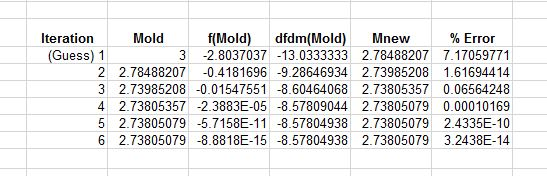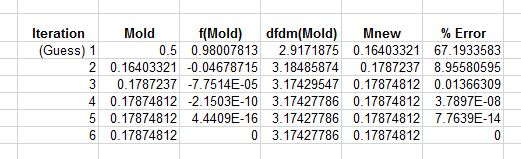Skip to content# Newton-Raphson Method

I was recently asked by a class to go over the Newton-Raphson method for solving non-linear equations. Specifically in this case it was to solve 1D gas dynamics equations. Here I will just do a brief overview of the method, and how its used. I will solve two cases, one where the derivative of the function is known, and one where the derivative of the function should be approximated.

## Contents

Here are the contents you’ll find in this post:

1. Introduction to the Newton-Raphson Method
2. Using the Method
1. Example 1 (derivative of the function is known)
• Calculating Mach number from critical area ratio (M from A/A*)
2. Example 2 (derivative of the function is unknown… or to annoying to derive)
• Calculating incident shock pressure ratio from diaphragm pressure ratio
3. Conclusions and Useful References

## Introduction to the Newton-Raphson Method

What is the Newton-Raphson method? Basically it is an iterative approach for solving the roots of functions. There are tons of these. You probably don’t need to know all of them (just pick a few that work for you!) Typically I stick to the Newton-Raphson method and the bisection method and I rarely have problems.

The Newton-Raphson method is used when you have some function f(x) and you want to find the value of the dependent variable (x) when the function equals zero. AKA you want to find the roots of the equation. If you have an initial guess at some point,$x_i$, the tangent can be extended to some point that crosses 0 at an easily calculable point$x_{i+1}$. This point gives an improved estimation of the root. The basics of the method come from the fact that the first derivative is equivalent to the slope and therefore if you know it, you can calculate the tangent intersection:$f'(x_i)=\frac{f(x_i)-0}{x_i-x_{i+1}}$  (1)

Which we can rearrange to get the “Newton-Raphson Formula”:$x_{i+1}=x_{i}-\frac{f(x_{i})}{\frac{df}{dx}(x_{i})}$  (2)

The method is depicted in the figure below:

Equation 2 pretty much sums up the method. If you start with a known derivative and function value$x_i$ you can calculate a new prediction$x_{i+1}$. By repeating this process (perform iterations) better and better approximations of the value for x are obtained.

## Using the Method

### Example 1 (derivative of the function is known):

Lets say for this example that we know a critical area ratio (let’s say A/A*=3.3, with$\gamma=1.4$) at a point in the flow field, and we want to use it calculate Mach number. For this we will solve the following equation:$\frac{A}{A^*}=\frac{1}{M}\left[\left( \frac{2}{\gamma+1}\right) \left(1 +\frac{\gamma -1}{2} M^2\right)\right]^{\frac{\gamma+1}{2\left(\gamma-1\right)}}$  (3)

This equation is equation for the critical area ratio for a given Mach number. Sometimes however the A/A* is known and the Mach number is desired. Let’s say A/A* =3.3 what is the Mach number? To do this, this equation can be easily solved using the Newton-Raphson approach.

Essentially the Newton-Raphson method is a root finding method. This means we are finding where an equation crosses zero. So how do we apply this to the problem above? We aren’t interested in where the equation above crosses zero, we are interested in where it crosses 3.3! Well it’s easy to change the above equation to give:$f(M)= \left[\left( \frac{2}{\gamma+1}\right) \left(1 +\frac{\gamma -1}{2} M^2\right)\right]^{\frac{\gamma+1}{2\left(\gamma-1\right)}} - M\frac{A}{A^*} = 0$  (4)

So now the problem is in a form that we can use the Newton-Raphson approach. In general, the approach is written as:$x_{new}=x_{old}-\frac{f(x_{old})}{\frac{df}{dx}(x_{old})}$  (5)

Here, f(x) is the equation that is to be solved (in our case Equation 4), x is the variable to be solved for (in our case Mach number) and df/dx is the derivative of the equation with respect to the unknown variable (in our case the derivative of Equation 4 with respect to Mach number).

So at this point we know f(M), but we must calculate the derivative of f(M). The result is the following:$\frac{df}{dM}(M)= \frac{A}{A^*}-(\frac{2}{\gamma+1})^{\frac{\gamma+1}{2\left(\gamma-1\right)}-1}M\left(1+\frac{(\gamma-1)M^2}{2}\right)^{\frac{\gamma+1}{2\left(\gamma-1\right)}-1}$  (6)

The next step is to apply the iterative algorithm. Essentially, you have to make an intelligent initial guess for the unknown variable (Mach number). In our case let’s make our first guess M=3. In our first step this is$M_{old}$. Using this value we can get a guess for$M_{new}$. Then at our next iteration$M_{new}$ becomes$M_{old}$ and this process is repeated until convergence ($M_{old} \approx M_{new}$).

The following is a table showing the convergence of this problem:So the Mach number for an A/A* of 3.3 is 2.738! But what if we had chosen a different starting point? Say… 0.5? Well then the answer looks like…Wait why is the answer different?? Dont forget! There are two solutions to this problem, a subsonic and a supersonic solution. When we started at M=3 we converged on the supersonic solution (M=2.73805). When we start at 0.5 we converged on the subsonic solution (M=0.17875).

### Example 2 (derivative df/dx is unknown… or too annoying to derive):

For example 2 we are again going to solve a simple 1D gas dynamics problem using the Newton-Raphson method. For this problem, let’s say that we are given a diaphragm pressure ratio for a shock tube ($P_4/P_1=40$). We want to solve for the pressure ratio that will exist  across the normal shock that is created when the diaphragm ruptures. For this problem see John and Keith (2006) for an excellent chapter on the shock-tube problem.

Basically this problem boils down to solving the following equation$\frac{P_4}{P_1}=\frac{P_2}{P_1}\left[1-\frac{(\gamma_4-1)(\frac{a_1}{a_4})(\frac{P_2}{P_1}-1)}{\sqrt{2\gamma_1}\sqrt{2\gamma_1+(\gamma_1+1)(\frac{P_2}{P_1}-1)}}\right]^{-\frac{2\gamma_4}{\gamma_4-1}}$          (7)

for a$\frac{P_2}{P_1}$ with a known shock tube pressure ratio$\frac{P_4}{P_1}$. Equation 5 is not the type of equation that is very attractive to differentiate, so in this example we will use a simple central different to approximate the derivative. Essentially what this means is that instead of directly calculating the derivative at each of our iterations, we will use a point slightly to the right and slightly to the left in order to approximate the slope.

First of all we rearrange (5) to give us an equation that equals zero (so that we can find its roots).  Demonstrating this is easier if we set our unknown$p=P_2/P_1$.$f(p)=\frac{P_4}{P_1}\left[1-\frac{(\gamma_4-1)(\frac{a_1}{a_4})(p-1)}{\sqrt{2\gamma_1}\sqrt{2\gamma_1+(\gamma_1+1)(p-1)}}\right]^{\frac{2\gamma_4}{\gamma_4-1}}-p=0$ (8)

For the Newton-Raphson the above equation would be solved using:$p_{new}=p_{old}-\frac{f(p_{old})}{\frac{df}{dp}(p_{old})}$  (9)

Since we do not know the derivative of f approximate it with a central difference (centered approximation of the local slope at that point):$\frac{df}{dp}(p_{old})=\frac{f(p_{old}+\Delta p) -f(p_{old}-\Delta p)} {2 \Delta p}$ (10)

so:$p_{new}=p_{old}-2\Delta p \frac{f(p_{old})}{f(p_{old}+\Delta p) -f(p_{old}-\Delta p)}$  (11)

With the above iterative equation, the problem is solved in the same fashion as Example 1. See the following table:Using Newton-Raphson we have now calculated that for a diaphragm pressure ratio of 40, the pressure ratio across the shock ($P_2/P_1$) should be 4.7726.

## Conclusion and Useful References

Hopefully this was helpful. The Newton-Raphson method is extremely useful. However be careful! There are some cases where this method doesn’t work very well. For example if an equation has multiple roots, your initial guess must be fairly close to the answer you are looking for or you could get the completely wrong root. It often helps to plot your function beforehand or after so that you know what it looks like, and it also helps to never blindly trust the answer. If it isn’t physical… it probably isn’t the one you were looking for.

Here are some useful references

 https://en.wikipedia.org/wiki/Newton%27s_method

 John, J. E. A., & Keith, T, G. (2006) Gas Dynamics, 3rd Edition, Pearson Prentice Hall

 Chapra, S. C., Canale, R. P. (2010) Numerical Methods for Engineers (6th Ed.), McGraw-Hill

Categories

Uncategorized